# Central exponents

(diff) ← Older revision | Latest revision (diff) | Newer revision → (diff)

of a linear system of ordinary differential equations

Quantities defined by the formulas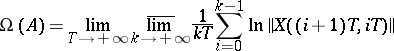(the upper central exponent) and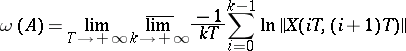(the lower central exponent); sometimes the lower central exponent is defined asHere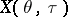is the Cauchy operator of the system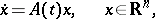(1)

where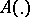is a mapping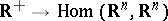that is summable on every interval. The central exponentsand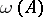may be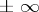; the inequalities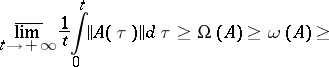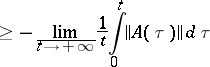hold, which imply that if the system (1) satisfies the condition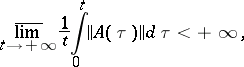then its central exponents are finite numbers. The central exponents are connected with the Lyapunov characteristic exponents (cf. Lyapunov characteristic exponent)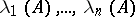and with the singular exponents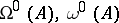by the inequalities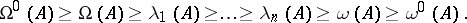For a system (1) with constant coefficientsthe central exponents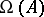andare equal, respectively, to the maximum and minimum of the real parts of the eigen values of. For a system (1) with periodic coefficients (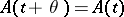for all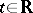and some,being the smallest period) the central exponentsandare equal, respectively, to the maximum and minimum of the logarithms of the moduli of the multipliers divided by the period.

Ifis an almost-periodic mapping (see Linear system of differential equations with almost-periodic coefficients), then the central exponents of (1) coincide with the singular exponents:(Bylov's theorem).

For every fixed system (1) the conditionis sufficient for the existence of a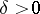such that for every system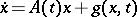satisfying the conditions of the existence and uniqueness theorem for the solution of the Cauchy problem and the condition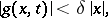the solution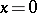is asymptotically stable (Vinograd's theorem). The condition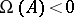in Vinograd's theorem is not only sufficient but also necessary. (The necessity remains valid when asymptotic stability is replaced by Lyapunov stability.)

The function(respectively,) on the spaceof the system (1) with bounded continuous coefficients (so thatis continuous and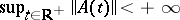), endowed with the metric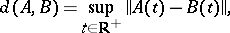is upper (respectively lower) semi-continuous, but neither of these functions is continuous everywhere. For every system (1), in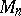one can find another system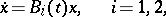(2)

arbitrarily close to it (in) such that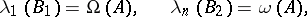where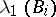and,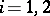, are the largest (highest) and the smallest (least) characteristic Lyapunov exponents of the system (2).

If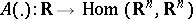is a uniformly-continuous mapping and if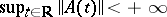, then for almost-every mapping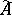(in the sense of every normalized invariant measure of shift dynamical systems (cf. Shift dynamical system),, concentrated on the closure of the trajectory of the point; the mappingsandare regarded as points of the space of the shift dynamical system) the upper (lower) central exponent of the system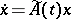is equal to the largest (smallest) characteristic Lyapunov exponent of this system: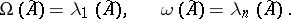Suppose that a dynamical system on a smooth closed manifold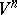is given by a smooth vector field. Then for almost-every point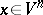(in the sense of every normalized invariant measure) the upper (lower) central exponent of the system of equations in variations along the trajectory ofcoincides with its largest (smallest) characteristic Lyapunov exponent. Generic properties of the central exponent (from the point of view of the Baire categories) have been studied, see .

How to Cite This Entry:
Central exponents. Encyclopedia of Mathematics. URL: http://encyclopediaofmath.org/index.php?title=Central_exponents&oldid=13711
This article was adapted from an original article by V.M. Millionshchikov (originator), which appeared in Encyclopedia of Mathematics - ISBN 1402006098. See original article